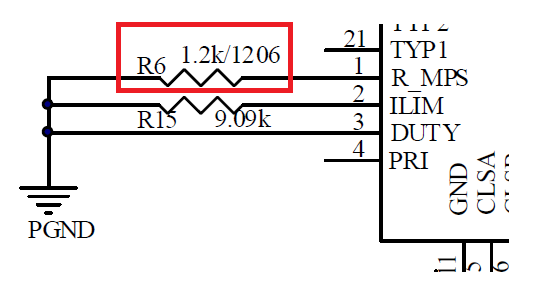# MP8030 : R_MPS current

R_MPS generates 24V pulse output when MPS function is triggered.
To maintain PSE power supply, MP8030 supports automatic maintain power signature and the current is programmed by resistor on R_MPS pin. If R6=1.2K is connected between R_MPS PIN and GND, then the current calculation is 24V/1.2K=20mA, right?Hi jasperc,

Yes, in theory. But to measure the actual current, I’d recommend using a DMM to measure the voltage across R6, then use I=V/R to determine the current.

Thanks,
Cindy